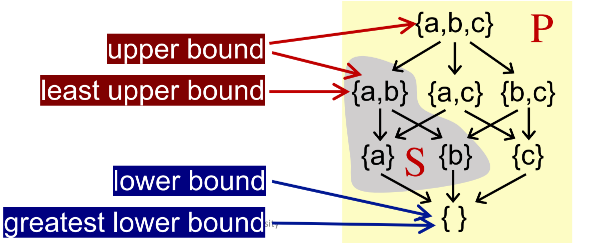# Static Program Analysis(Foundations)

## Partial Order

We define poset as a pari (P, ⊑) where ⊑ is a binary relation that defines a partial ordering over P and ⊑ has the following properties:

Reflexivity(自反性)：∀x ∈ P, x ⊑ x

Antisymmetry(反对称性): ∀x, y ∈ P, x ⊑ y ∧ y ⊑ x ⟹ x = y

Transitivity(传递性): ∀x, y, z ∈ P, x ⊑ y ∧ y ⊑ z ⟹ x ⊑ z

Partial means for a pair of set elements in P,they could be incomparable; in other words, not necessary that every pair of set elements must satisfy the ordeing ⊑

## Upper and Lower Bounds

Given a poset (P, ⊑) and its subset S that S ⊆ P, we say that

u ∈ P is an upper bound of S, if ∀x ∈ S, x ⊑ u. Similarly,

l ∈ P is an lower bound of S, if ∀x ∈ S, l ⊑ x.

We define the least upper bound(lub or join) of S, written ⊔S, if for every upper bound of S, say u, ⊔S ⊑ u.

Similarly, We define the greatest lower bound (glb or meet) of S, written ⊓S, is for every lower bound of S,say l, l ⊑ ⊓S.Some Properties:

• Not every poset has lub or glb
• But if a poset has lub or glb, it will be unique

## Lattice

Given a poset (P, ⊑), ∀a, b ∈ P, if a ⊔ b and a ⊓ b exist, then (P, ⊑) is called a lattice

(A poset is a lattice if every pair of its elements has a least upper bound and a greatest lower bound)

### Complete Lattice

All subsets of a lattice have a least upper bound and a greatest lower bound

Every finite lattice is a complete lattice. But complete lattice is not necessarily finite

### Product Lattice

Given lattices L1 = (P 1 , ⊑1 ), L2 = (P 2 , ⊑2 ), …, Ln = (P n , ⊑n ), if for all i,(P i , ⊑i ) has ⊔i (least upper bound) and ⊓i (greatest lower bound), then we can have a product lattice Ln = (P, ⊑) that is defined by:
• P = P 1 × … × P n
• (x 1 , …, xn ) ⊑ (y 1 , …, yn ) ⟺ (x 1 ⊑ y1 ) ∧ … ∧ (xn ⊑ yn )
• (x 1 , …, xn ) ⊔ (y 1 , …, yn ) = (x 1 ⊔1 y1 , …, xn ⊔n yn )
• (x 1 , …, xn ) ⊓ (y 1 , …, yn ) = (x 1 ⊓1 y1 , …, xn ⊓n yn )

A product lattice is a lattice

If a product lattice L is a product of complete lattices,then L is also complete

## Fixed-Point Theorem

Given a complete lattice(L,⊑) if

1. f: L -> L is monotonic (单调)
2. L is finite (有界)

then the least fixed point of f can be found by iterating f(⊥), f(f(⊥)), …, f (… (⊥)…) until a fixed point is reached

and the greatest fixed point of f can be found by iterating f(T), f(f(T)), …, f(…f (T )…) until a fixed point is reached